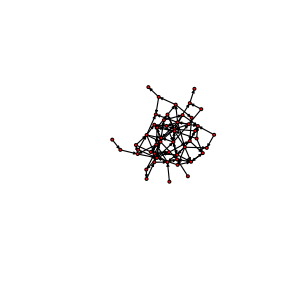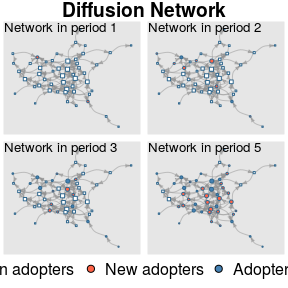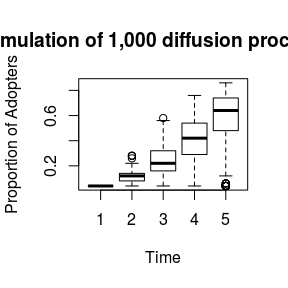# A simple simulation example

## Step 1: Loading the packages

library(netdiffuseR)
library(sna)

## Step 2: Random network (for input)

set.seed(8826)

# Simulating a small world (10, 3) with pr = .3
net <- rgraph_ws(50, 3, .3)

# A bit more of rewiring
net <- rewire_graph(net, p=3, both.ends = TRUE)

Taking a look at it using sna

# Visualizing with
gplot(as.matrix(net))# Random diffusion with a fixed threshold of 1, simulating 5 time points
mydiffnet <- rdiffnet(
seed.graph     = net,                    # The network we just created
threshold.dist = 1,                      # Fixed threshold of 1
t              = 5,                      # 5 time points
rewire         = FALSE,                  # No rewire (defaults TRUE)
exposure.args  = list(normalized=FALSE), # Exposure to be computed unnormalized
# so we use counts instead
seed.nodes     = "random"                # Random set of initial adopters
)

# Looking at the diffusion process
plot_diffnet(mydiffnet)# Some summary stats
summary(mydiffnet)
## Diffusion network summary statistics
## Name     : A diffusion network
## Behavior : Random contagion
## -----------------------------------------------------------------------------
##  Period   Adopters   Cum Adopt. (%)   Hazard Rate   Density   Moran's I (sd)
## -------- ---------- ---------------- ------------- --------- ----------------
##        1          2         2 (0.04)             -      0.04 -0.04 (0.03)
##        2          7         9 (0.18)          0.15      0.04  0.05 (0.03) **
##        3          9        18 (0.36)          0.22      0.04  0.05 (0.04) *
##        4         14        32 (0.64)          0.44      0.04  0.08 (0.04) ***
##        5          9        41 (0.82)          0.50      0.04  0.24 (0.03) ***
## -----------------------------------------------------------------------------
##  Left censoring  : 0.04 (2)
##  Right centoring : 0.18 (9)
##  # of nodes      : 50
##
##  Moran's I was computed on contemporaneous autocorrelation using 1/geodesic
##  values. Significane levels  *** <= .01, ** <= .05, * <= .1.

We can actually go further and run multiple simulations instead so that we can get a confidence interval in the proportion of adopters

set.seed(871)
mydiffnet <- rdiffnet_multiple(
statistic      = function(n) cumulative_adopt_count(n)["prop",],
R              = 1000,
stop.no.diff   = FALSE,                  # This option allows us to continue
# The simulation process, even if there
# is no adoption.
seed.graph     = net,                    # The network we just created
threshold.dist = 1,                      # Fixed threshold of 1
t              = 5,                      # 5 time points
rewire         = FALSE,                  # No rewire (defaults TRUE)
exposure.args  = list(normalized=FALSE), # Exposure to be computed unnormalized
# so we use counts instead
seed.nodes     = "random"                # Random set of initial adopters
)

# Looking at the diffusion process
boxplot(
t(mydiffnet),
xlab = "Time",
ylab = "Proportion of Adopters",
main = "Simulation of 1,000 diffusion processes"
)